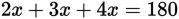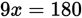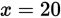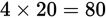# SAT Math Multiple Choice Question 952: Answer and Explanation

### Test Information

Question: 952

4. The angles of a triangle are in the ratio 2:3:4. What is the degree measure of thelargest angle?

• A. 40
• B. 80
• C. 90
• D. 120

Getting to the Answer: Because the angles are in the ratio 2:3:4, use 2x, 3x, and 4x to represent their measures. This means, or when simplified,, which yields. The largest angle has measure 4x, or, making (B) the correct answer.